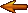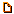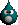# Topic: Big O notation algorithms (Page 1 of 1)binary
Paranoid (IV) Inmate

From: Under the Bridge
Insane since: Nov 2002posted 11-28-2006 13:05

Hi All,

Pls some help over here....1)Suppose algorithm A requires nlog2n to sort n items and B requires (n^2)/4 to sort the n items for which n is algorithm B superior to algorithm A

2) Show that n>=4(n/4)log(n/2) = (n/4)log n

Thanks

~Sig coming soon~

WarMage

From: Rochester, New York, USA
Insane since: May 2000posted 11-28-2006 23:13

1. You should use a graphic calculator to graph these two functions.

You will find that there are 2 intersection points:

n = 0.8

and

n = 3.25.

n < 0.8 n*log(2*n) is faster
n = 0.8 they are the same
n > 0.8 and n < 3.25 (x^2)/4 is faster
n = 3.25 they are the same
n > 3.25 n*log(2*n) is faster

2. This is a false statement.
When n = 4,

(n/4)log(n/2) = log(2) ~= 0.3

and

(n/4)log(n) = log(4) ~= 0.6

This 0.3 <> 0.6

However, if you can make use of a rule which allows you eliminate constants. You will have:
(n/4)log(n/2) =
(n/4)(log(n) + log(2)) =
(n/4)(log(n) - 0.3)

Dan @ Code Town

Slime

From: Massachusetts, USA
Insane since: Mar 2000posted 12-05-2006 10:03

This is a little late, but...

Your topic mentions "Big O notation," yet your post doesn't. This scares me a little because I don't think you realize the difference between "O(expression)" and just "expression".

Suppose algorithm A requires O(nlog2n) to sort n items and B requires O((n^2)/4) to sort the n items. For which n is algorithm B superior to algorithm A?

There is no answer to this question. The reason is that Big-O-notation describes the runtime of an algorithm only as a proportion relative to itself. For instance, saying that an algorithm is O(n) only says that when you make n, say, twice as large, the algorithm takes twice the time to run. This could mean that the algorithm goes from 1 second to 2 seconds, or from 1 year to 2 years - there's no way to know from just the O(n).

So, you know something about algorithm A, and you know something about algorithm B, but you don't know anything about them relative to each other. You don't know for specifically which n A is faster than B; you can only say that as n gets bigger and bigger, algorithm A will "eventually" be faster than algorithm B, because its runtime doesn't increase as quickly relative to n. This might happen when n is 10 or when n is 1000000.

Your second question also worries me, because I'm worried that you're actually talking about big-O-notation as compared to big-Omega and big-Theta notation. The relationships between these notations can be *compared* to simple inequalities, but because of the subtleties I pointed out above, they are not equivalent!

Perhaps you meant:

Show that n is Omega( 4(n/4)log(n/2) ) and that 4(n/4)log(n/2) is Theta( (n/4)log n )

In order to prove these, you will need to use the actual definitions of the notations. Specifically, to show that n is Omega( 4(n/4)log(n/2) ), you need to show that there are some values of n0 and M for which, when n > n0, n > M * 4(n/4)log(n/2). This is a little complex and may take some time to fully understand.

Again, this is probably too late to help you with whatever assignment you were working on, but hopefully you'll find the actual knowledge useful...